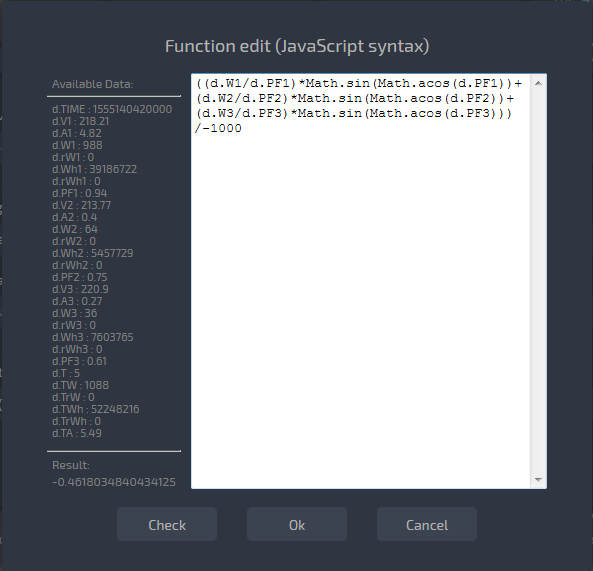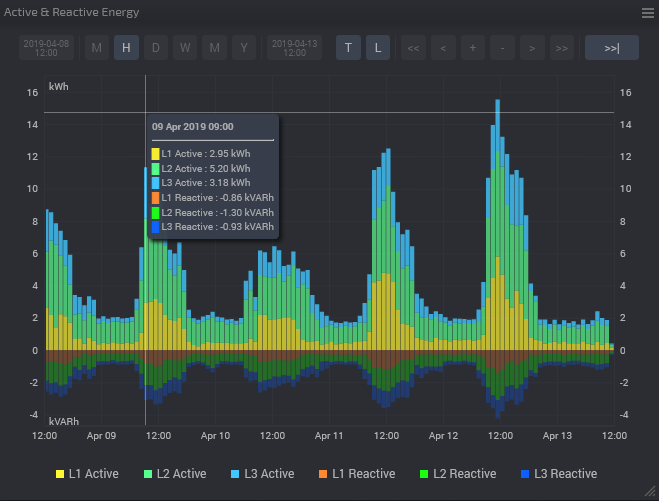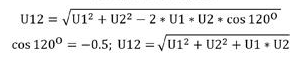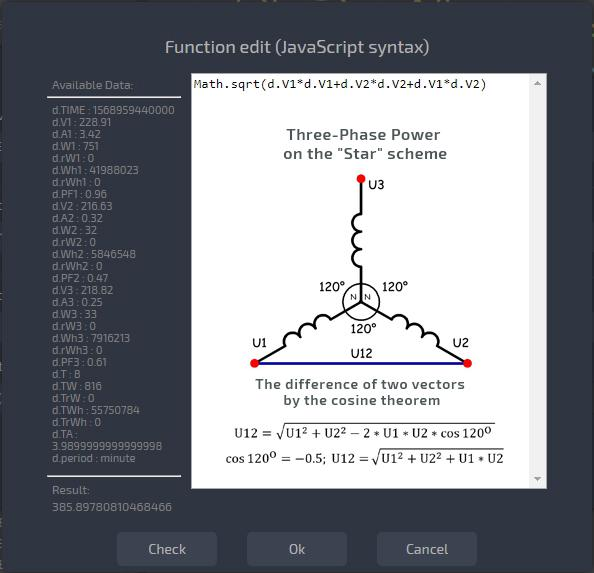# Reactive Power [VAR] and Reactive Energy [VARh]. Line Voltage.

#### Reactive power [VAR] and energy [VARh]

Reactive power and energy can be obtained by calculation.

But first, it is important to understand how parameters are calculated at different time intervals.
It is particularly important to understand the principle of calculation for parameter [Power] when it becomes from electrical power into electrical energy.

 smart-MAC parameters, symbol Real-Time and Minute interval Hour, Daily, Weekly, Monthly intervals Voltage, V Voltage [V] Arithmetic average of Voltage [V] Current, A Current [A] Arithmetic average of Current [A] Power, W Instant Power [W] Energy for the period [Wh] Power Factor, PF Power Factor (cosФ) Arithmetic average of Power Factor Energy, Wh Cumulative energy meter [Wh] Cumulative energy meter [Wh]

Energy meter smart-MAIC measures:

-Active Power [Power]

-Power Factor (cosF) [PF]

That's enough to get the calculated Apparent Power and Reactive Power. Remember physics course:)

```Active Power = Apparent Power * cosФ
```

from here:

```Apparent Power = Active Power / cosФ
// it is important in our case to divide the Active Power on the Power Factor (cosФ).
```
```Reactive Power = Apparent Power * sinФ
where angle Ф = arcos (cosФ)```
##### To display the reactive power and energy in digital or charts Widget:

- open the Widgets settings;
- in section "Arithmetic operation" select the function f(x);
- click on the "Edit" to open the window for editing complex functions.
On the left side you can see a list of the data and their symbol for use in the formula. When you build formulas, you can use any arithmetic and mathematical functions of the JavaScript language. To test the function, use the button "Check" and test the values of variables.

The formula for the calculation of the Reactive Power for the first phase of three-phase energy meter will look like:

```(d.W1 / d.PF1) * Math.sin(Math.acos( d.PF1 )) ||0
// the operator "|| 0" returns Zero if Power Factor is zero, for example, if one line is disabled
```

The formula for the calculation of the Total Reactive Power in kVAR of three-phase energy meter will look like:

```(((d.W1/d.PF1)*Math.sin( Math.acos(d.PF1) )||0) + ((d.W2/d.PF2)*Math.sin( Math.acos(d.PF2) )||0) + ((d.W3/d.PF3)*Math.sin( Math.acos(d.PF3) )||0))/-1000
// to multiplication by a negative will build the chart in the down axles
```Note:

- for a minute and Real-Time intervals,  it will be Reactive Power [VAR]

- for hour, day and above intervals, it will be Reactive Energy [VARh]

- computations are averaged for the period because the calculation used average Power Factor for the period (cosФ)

#### Line Voltage.

Line voltage is the voltage measured between any two lines in a three-phase circuit.

To control the line voltage in a three-phase network you can use the calculated values ​​from the smart-MAIC D103 energy meter.
The voltage in a Three-Phase Power connected on the "star" scheme is three vectors with a length of 220V and an angle between vectors of 120° (360°/3). Then the line voltage is the difference of two vectors, which is easy to find by the cosine theorem.

For example, the line voltage between the first and second phases:With the powerful tool f(x) we can write in the widget settings on the Dashboard this formula:

`Math.sqrt(d.V1*d.V1+d.V2*d.V2+d.V1*d.V2)`